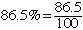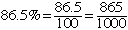Subject: Middle school math I need help with these math questions: How does one convert a percent to a fraction when the percent has a decimal (example-86.5%)? Andrew Connell Hi Andrew, PerCENT means per 100 soNow multiply numerator and denominator get 10 to getFinally you should write the fraction in lowest terms. Cheers, Penny

Go to Math Central